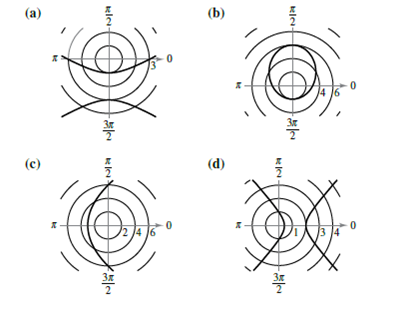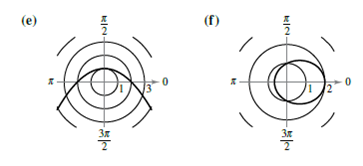Chapter 10.6, Problem 8E

Chapter
Section
Textbook Problem

# Matching In Exercises 7-12, match the polar equation with its correct graph. [The graphs are labeled (a), (b), (c), (d>, (e), and f).]r = 1 2 − cos θ

To determine

The correct match of the given polar equation with its correct graph from the options given.

Explanation

Given: Given options for graphs are labelled as (a), (b), (c), (d), (e) and (f) as shown below:

Calculation: The given equation is r=22cosθ.

Rewrite the equation as

r=22cosθ=11(1/2)cosθ

So, the graph of the given equation is an ellipse with e=12

### Still sussing out bartleby?

Check out a sample textbook solution.

See a sample solution

#### The Solution to Your Study Problems

Bartleby provides explanations to thousands of textbook problems written by our experts, many with advanced degrees!

Get Started

#### Solve each inequality for x. 56. (a) 1 e3x1 2 (b) 1 2 ln x 3

Single Variable Calculus: Early Transcendentals, Volume I

#### Simplify each of the following so that only positive exponents remain.

Mathematical Applications for the Management, Life, and Social Sciences

#### Evaluate the indefinite integral. sinxxdx

Single Variable Calculus: Early Transcendentals

#### The directrix of the conic given by is:

Study Guide for Stewart's Multivariable Calculus, 8th

#### True or False: By the Integral Test, converges.

Study Guide for Stewart's Single Variable Calculus: Early Transcendentals, 8th